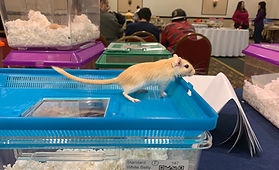top of page

# Males92

First

average rating is 4.6 out of 5146

First

average rating is 4.8 out of 524

First BOS

average rating is 4.4 out of 558

First

average rating is 1 out of 583

First BOS

average rating is 4.4 out of 58

First

average rating is 5 out of 543

First

average rating is 5 out of 5108

First

average rating is 4.7 out of 575

First

average rating is 4.8 out of 516

First

average rating is 5 out of 552

First

average rating is 3.9 out of 5104

First

average rating is 4.2 out of 591

First BOS

average rating is 4.5 out of 518

First BOS

average rating is 4.2 out of 548

First BOS

average rating is 4.1 out of 5127

First

average rating is 4.8 out of 586

First

average rating is 4.4 out of 529

First

average rating is 4.5 out of 554

First BOS

average rating is 4.3 out of 5139

First BOS

average rating is 4.6 out of 52

First BOS

average rating is 4.6 out of 536

First

average rating is 4.8 out of 5147

First BOS

average rating is 4.7 out of 5126

First BOS

average rating is 4.6 out of 5

bottom of page